# Solution assignment 05 Exponential functions and graphs

### Assignment 5

Draw the graph of the function: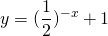What is the formula of the asymptote?

### Solution

We write: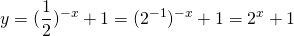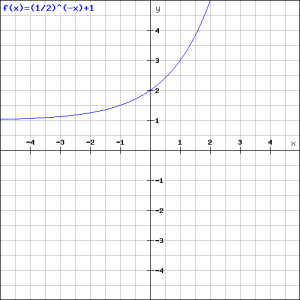We want to draw the graph of this function and start with the exponential function and add. The result is in the figure.
The asymptote of the exponential function is the-as, i.e.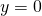and thus the de asymptote of the graph as a whole is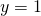.

0